Curvature

Thughout this section, we use the unique "Levi-Civita" connection. First, consider the metric defined in the previous section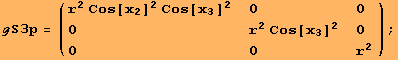In the following, we describe how to obtain the Riemannian curvature (1,3)-tensor field. In fact, the command ΜR does the job.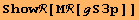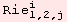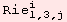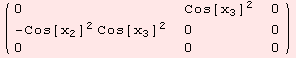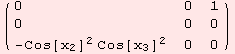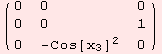The (0,2)-Ricci curvature tensor field computes as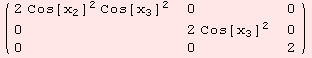Many more examples, will follow in later sections. At this place, we point to the feature of using ShowRFunc for more extensive terms.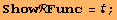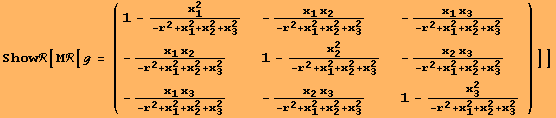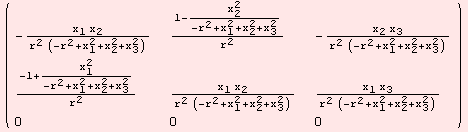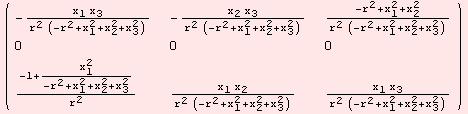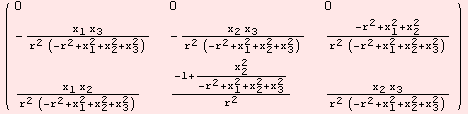... and the Ricci curvature.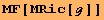Created by Mathematica  (December 22, 2006)